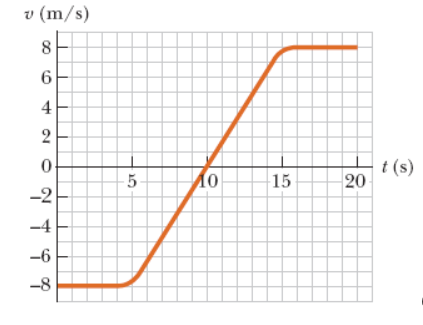Question
1 views

The velocity vs. time graph for an object moving along a straight path is shown in the figure below.

(i) Find the average acceleration of this object during the following time intervals.

(a)    0 s to 5.0 s

(b)    5.0 s to 15 s

(c)    0 s to 20 s

(ii) Find the instantaneous accelerations at the following times.

(a)    2.0 s

(b)    10 s

(c)    18 shelp_outlineImage Transcriptionclosev (m/s) 4 2 0 t(s) 20 -5 Ло 15 -2 -4 -6 -8 00 fullscreen
check_circle

star
star
star
star
star
1 Rating
Step 1

According to our instructions we are supposed to answer only three sub parts of question.

If any specific sub part is to be answered please mention while posting the question

Step 2

From the given graph we can observe that the velocity of the object is constant (i.e. -8 m/s)

from “t = 0 s” to “t = 5 s” hence we can say that acceleration of is “zero” in this time period.

Step 3

We can observe from the graph, that in the time interval “t = 5 s” to “t = 15 s” the velocity of th...

### Want to see the full answer?

See Solution

#### Want to see this answer and more?

Solutions are written by subject experts who are available 24/7. Questions are typically answered within 1 hour.*

See Solution
*Response times may vary by subject and question.
Tagged in

### Kinematics